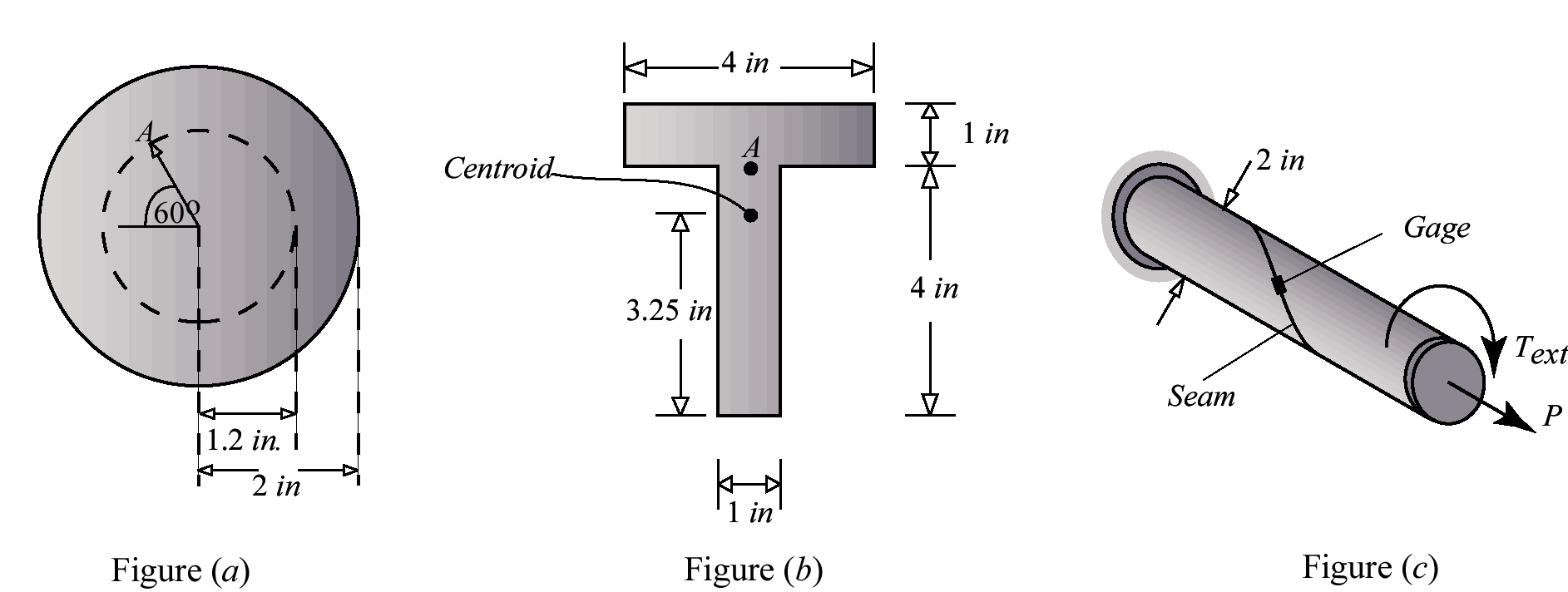# 3.4 – BASIC STRUCTURAL MEMBERS

In the questions below, choose the best answer considering tension as positive and compression as negative. Use modulus of elasticity of 30,000 ksi and Poisson’s ratio of 0.25. Report all answers to three significant figures. The seam is 30o to the axis of the shaft.Name Email
1. In Figure (a), the torsional shear strain at point A on the solid circular cross-section was found to be 0.0018. The maximum shear strain on the cross-section is
2. In Figure (a), the torsional shear stress at point A on the solid circular cross-section was found to be 12 ksi. The maximum shear stress on the cross-section is
3. In Figure (a), the maximum torsional shear strain on the solid circular cross-section was found to be 0.0015. The torsional shear stress at point A is
4. In Figure (b), the bending normal strain at point A on the cross-section of a beam was found to be 0.00015. The maximum bending normal strain in the cross-section is
5. In Figure (b), the bending normal stress at point A on the cross-section of a beam was found to be 1.5 ksi (T). The maximum bending normal stress in the cross-section is
6. In Figure (b), the bending normal strain at point A on the cross-section of a beam was found to be 0.00015. The maximum bending normal stress in the cross-section is
7. In Figure (c), for P = 9π kips and Text = 0 the strain recorded by the strain gage is
8. In Figure (c), for P = 0 and Text = 12π in-kips the strain recorded by the strain gage is
9. In Figure (c), for P = 9π kips and Text = 0 the normal stress on the seam plane at A is
10. In Figure (c), for P = 0 and Text = 12π, the normal stress on the seam plane at A is